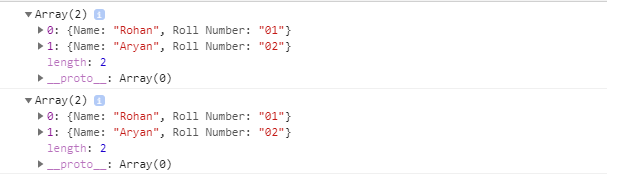# How to convert CSV string file to a 2D array of objects using JavaScript ?

• Last Updated : 11 Apr, 2022

A CSV is a comma-separated values file with a .csv extension, which allows data to be saved in a tabular format.

In this article, we will learn to convert the data of a CSV string to a 2D array of objects, where the first row of the string is the title row using JavaScript.

Given a comma-separated values (CSV) string to a 2D array, using JS.f

```Input: 'Name,Roll Number\nRohan,01\nAryan,02'

Output:  [
{Name: "Rohan", Roll Number: "01"},
{Name: "Aryan", Roll Number: "02"}
]
// With delimiter ;

Input: 'Name;Roll Number\nRohan;01\nAryan;02'

Output:    [
{Name: "Rohan", Roll Number: "01"},
{Name: "Aryan", Roll Number: "02"}
]    ```

We must know some array and string prototype functions that will be helpful in this regard.

indexOf function: The String.prototype.indexOf() function finds the index of the first occurrence of the argument string in the given string and the value returned is in 0 based index.

Example:

```str = 'How\nare\nyou?'

str.indexOf('\n');```

Output:

`3`

Slice function: The Array.prototype.slice() method returns a new array containing a portion of the array on which it is implemented and the original array remains the same.

Example:

`['a','b','c','d'].slice(1)`

Output:

` ['b','c','d']`

Map function: The Array.prototype.map() method returns a new array with the results of calling a provided function on every element.

Example:

```arr = [2, 4, 8, 16]

// Dividing each element of the array by 2
newArr = arr.map( item => item/2) ```

Output:

`[1, 2, 4, 8]`

Split function: The String.prototype.split() method is used to split the given string into an array of strings by separating it into substrings using a specified separator provided in the argument.

Example:

```str = "Geeks for Geeks"

// Split the array when ' ' is located
arr = str.split(' ');```

Output:

`[ 'Geeks', 'for', 'Geeks' ]`

Reduce function: The Array.prototype.reduce() method in JavaScript is used to reduce the array to a single value and executes a provided function for each element of the array from left to right and the return value of the function is stored in an accumulator.

Example:

``` arr = [2,4,6,8]

// Here 0 is the initial value of the accumulator
// while traversing, currentValue has been added

arr.reduce(function(accumulator,currentValue){
return accumulator+currentValue;
},0)```

Output:

`20`

Approach:

• The JavaScript string slice() method extracts parts of a string and returns the extracted parts in a new string taking ‘\n’ as the first occurrence.
• Data Values are stored using “\n” as the delimiter.
• JavaScript map() function will iterate over all values of title values array and append each object at the end of the array
• The “storeKeyValue” variable is used to store each key with its respective values.

Example:

## Javascript

 ``

Output:My Personal Notes arrow_drop_up